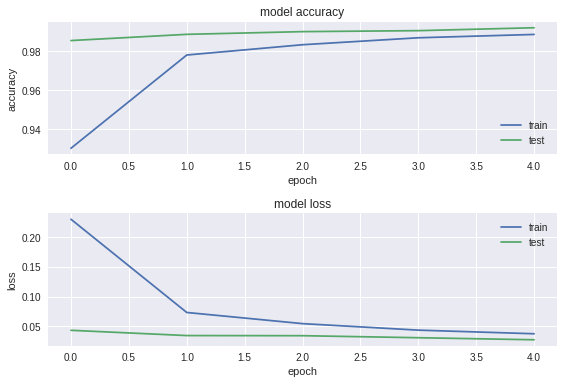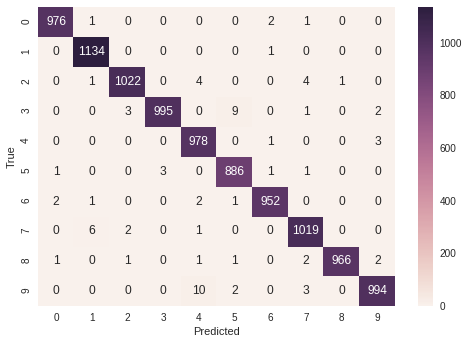2. Implementing a Neural Network¶

2.1 Hyperparameters¶

In :
# Hyperparameters
training_epochs = 5 # Total number of training epochs
learning_rate = 0.03 # The learning rate

2.2 Creating a model¶

Conv2D - This layer creates a convolution kernel that is convolved with the layer input to produce a tensor of outputs.

BatchNormalization - Normalize the activations of the previous layer at each batch, i.e. applies a transformation that maintains the mean activation close to 0 and the activation standard deviation close to 1.

Max pooling is a sample-based discretization process. The objective is to down-sample an input representation (image, hidden-layer output matrix, etc.), reducing its dimensionality and allowing for assumptions to be made about features contained in the sub-regions binned.

Dropout is a technique used to improve over-fit on neural networks, you should use Dropout along with other techniques like L2 Regularization. Basically during training some of neurons on a particular layer will be deactivated. This improve generalization because force your layer to learn with different neurons the same "concept". During the prediction phase the dropout is deactivated.

Flatten - Flattens the input. Does not affect the batch size.

To make this work in keras we need to compile a model. An important choice to make is the loss function. We use the categorical_crossentropy loss because it measures the probability error in discrete classification tasks in which the classes are mutually exclusive (each entry is in exactly one class).

In :
# create a model
def create_model():
model = Sequential()

model.add(Conv2D(filters = 16, kernel_size = (3,3), activation='relu',input_shape = (28,28,1)))
model.add(Conv2D(filters = 16, kernel_size = (3,3), activation='relu'))

model.add(Conv2D(filters = 32, kernel_size = (3,3), activation='relu'))
model.add(Conv2D(filters = 32, kernel_size = (3,3), activation='relu'))

# Compile a model
return model

model = create_model()
model.summary()
WARNING:tensorflow:From /usr/local/lib/python3.6/dist-packages/tensorflow/python/framework/op_def_library.py:263: colocate_with (from tensorflow.python.framework.ops) is deprecated and will be removed in a future version.
Instructions for updating:
Colocations handled automatically by placer.
WARNING:tensorflow:From /usr/local/lib/python3.6/dist-packages/keras/backend/tensorflow_backend.py:3445: calling dropout (from tensorflow.python.ops.nn_ops) with keep_prob is deprecated and will be removed in a future version.
Instructions for updating:
Please use rate instead of keep_prob. Rate should be set to rate = 1 - keep_prob.
_________________________________________________________________
Layer (type)                 Output Shape              Param #
=================================================================
conv2d_1 (Conv2D)            (None, 26, 26, 16)        160
_________________________________________________________________
batch_normalization_1 (Batch (None, 26, 26, 16)        64
_________________________________________________________________
conv2d_2 (Conv2D)            (None, 24, 24, 16)        2320
_________________________________________________________________
batch_normalization_2 (Batch (None, 24, 24, 16)        64
_________________________________________________________________
max_pooling2d_1 (MaxPooling2 (None, 12, 12, 16)        0
_________________________________________________________________
dropout_1 (Dropout)          (None, 12, 12, 16)        0
_________________________________________________________________
conv2d_3 (Conv2D)            (None, 10, 10, 32)        4640
_________________________________________________________________
batch_normalization_3 (Batch (None, 10, 10, 32)        128
_________________________________________________________________
conv2d_4 (Conv2D)            (None, 8, 8, 32)          9248
_________________________________________________________________
batch_normalization_4 (Batch (None, 8, 8, 32)          128
_________________________________________________________________
max_pooling2d_2 (MaxPooling2 (None, 4, 4, 32)          0
_________________________________________________________________
dropout_2 (Dropout)          (None, 4, 4, 32)          0
_________________________________________________________________
flatten_1 (Flatten)          (None, 512)               0
_________________________________________________________________
dense_1 (Dense)              (None, 512)               262656
_________________________________________________________________
dropout_3 (Dropout)          (None, 512)               0
_________________________________________________________________
dense_2 (Dense)              (None, 1024)              525312
_________________________________________________________________
dropout_4 (Dropout)          (None, 1024)              0
_________________________________________________________________
dense_3 (Dense)              (None, 10)                10250
=================================================================
Total params: 814,970
Trainable params: 814,778
Non-trainable params: 192
_________________________________________________________________

2.3 Train the model¶

Let's trains the model for a given number of epochs.

In :
results = model.fit(
X_train, y_train,
epochs= training_epochs,
batch_size = 128,
validation_data = (X_test, y_test),
verbose = 2
)
WARNING:tensorflow:From /usr/local/lib/python3.6/dist-packages/tensorflow/python/ops/math_ops.py:3066: to_int32 (from tensorflow.python.ops.math_ops) is deprecated and will be removed in a future version.
Instructions for updating:
Train on 60000 samples, validate on 10000 samples
Epoch 1/5
- 12s - loss: 0.2299 - acc: 0.9301 - val_loss: 0.0433 - val_acc: 0.9856
Epoch 2/5
- 9s - loss: 0.0732 - acc: 0.9781 - val_loss: 0.0344 - val_acc: 0.9888
Epoch 3/5
- 9s - loss: 0.0545 - acc: 0.9835 - val_loss: 0.0342 - val_acc: 0.9902
Epoch 4/5
- 9s - loss: 0.0435 - acc: 0.9870 - val_loss: 0.0308 - val_acc: 0.9907
Epoch 5/5
- 9s - loss: 0.0375 - acc: 0.9887 - val_loss: 0.0274 - val_acc: 0.9922

2.4 Test the model¶

Model can generate output predictions for the input samples.

In :
prediction_values = model.predict_classes(X_test)

2.5 Accuracy¶

Test-Accuracy :

In :
print("Test-Accuracy:","%.2f%%" % (np.mean(results.history["val_acc"])*100))
Test-Accuracy: 98.95%

2.6 Evaluate the model to see the accuracy¶

Now we can check the accuracy of our model

In :
print("Evaluating on training set...")
(loss, accuracy) = model.evaluate(X_train,y_train)
print("loss={:.4f}, accuracy: {:.4f}%".format(loss,accuracy * 100))

print("Evaluating on testing set...")
(loss, accuracy) = model.evaluate(X_test, y_test)
print("loss={:.4f}, accuracy: {:.4f}%".format(loss,accuracy * 100))
Evaluating on training set...
60000/60000 [==============================] - 5s 91us/step
loss=0.0194, accuracy: 99.4000%
Evaluating on testing set...
10000/10000 [==============================] - 1s 101us/step
loss=0.0274, accuracy: 99.2200%

2.7 Summarize history for accuracy and loss¶

In :
# summarize history for accuracy
plt.subplot(211)
plt.plot(results.history['acc'])
plt.plot(results.history['val_acc'])
plt.title('model accuracy')
plt.ylabel('accuracy')
plt.xlabel('epoch')
plt.legend(['train', 'test'], loc='down right')

# summarize history for loss
plt.subplot(212)
plt.plot(results.history['loss'])
plt.plot(results.history['val_loss'])
plt.title('model loss')
plt.ylabel('loss')
plt.xlabel('epoch')
plt.legend(['train', 'test'], loc='upper right')
plt.tight_layout()

max_loss = np.max(results.history['loss'])
min_loss = np.min(results.history['loss'])
print("Maximum Loss : {:.4f}".format(max_loss))
print("Minimum Loss : {:.4f}".format(min_loss))
print("Loss difference : {:.4f}".format((max_loss - min_loss)))
Maximum Loss : 0.2299
Minimum Loss : 0.0375
Loss difference : 0.19252.8 Confusion matrix¶

In :
Y_true = np.argmax(y_test,axis = 1)
confusion_mtx = confusion_matrix(Y_true, prediction_values)
sns.heatmap(confusion_mtx, annot=True, fmt="d")
plt.ylabel('True')
plt.xlabel('Predicted')
Out:
Text(0.5, 28.5, 'Predicted')2.8 Save a model to JSON and HDF5¶

In :
model_json = model.to_json()
with open("CNN_model_Keras_digits_recoginition.json", "w") as json_file:
json_file.write(model_json)
# save weights to HDF5
model.save_weights("CNN_model_Keras_digits_recoginition.h5")